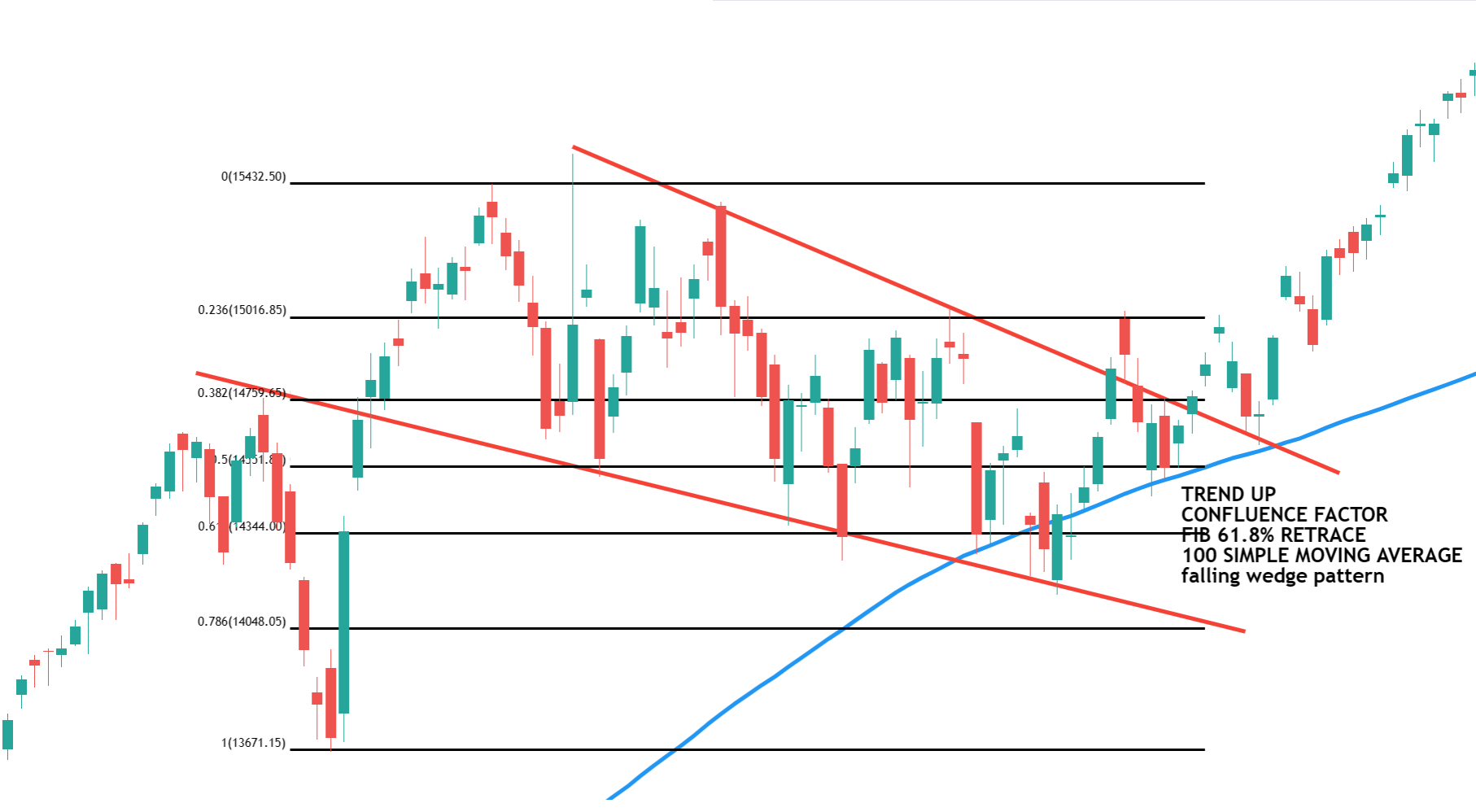# Fibonacci Trading Strategy using Confluence Factor

## Fibonacci Trading Strategy using Confluence Factor

1. Why does Fibonacci Work?
2. Fibonacci Trendline Confluence Trading Strategy
3. Confluence of Fibonacci with Price support or resistance or supply demand zone confluence trading strategy
4. Confluence of Fibonacci with moving average confluence trading strategy
5. Fibonacci with Multiple Confluence Trading Strategy
##### Fibonacci Trading Strategy using Confluence Factor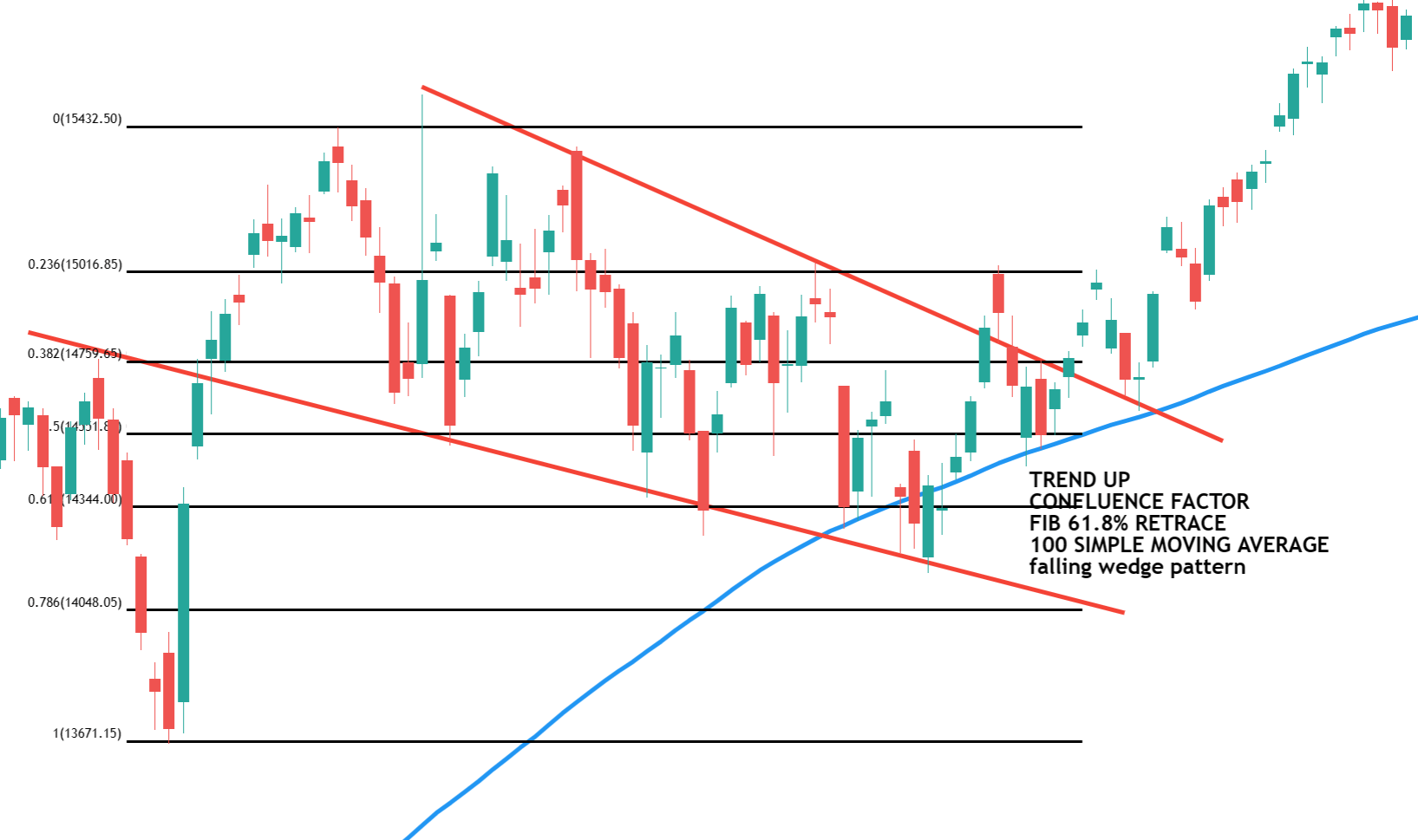##### Principles of Market Trends

As you know price action has a zig-zag form

1. Movements with the trend are called “impulses/extensions”.
2. Movements against the trend are called “correction/retracement”.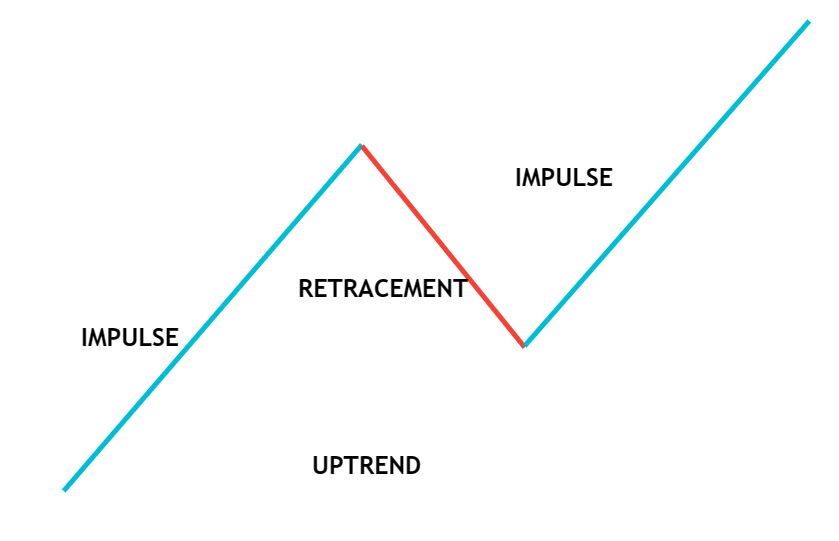##### Fibonacci RETRACEMENT

After beginning a new trend direction, the price will retrace before continuing in the trend’s direction. Therefore, we use the Fibonacci tool to identify the following probable support and resistance levels. The maximum level at which retracement is likely to occur is predicted by the Fibonacci retracement level. Traders have a good opportunity to start new trades in the trend’s direction at these retracement levels.

#####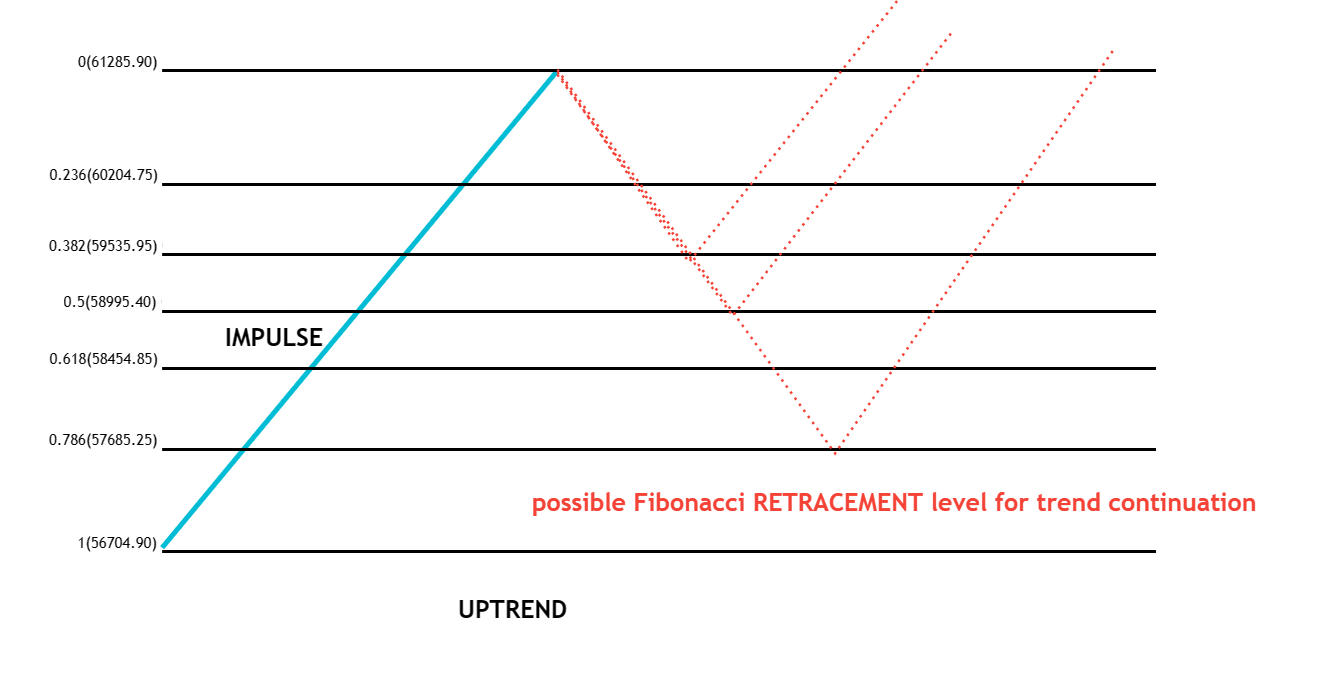Fibonacci EXTENSION

Traders can use Fibonacci extensions as a tool to set profit targets or predict how far a market may rise when a retracement is complete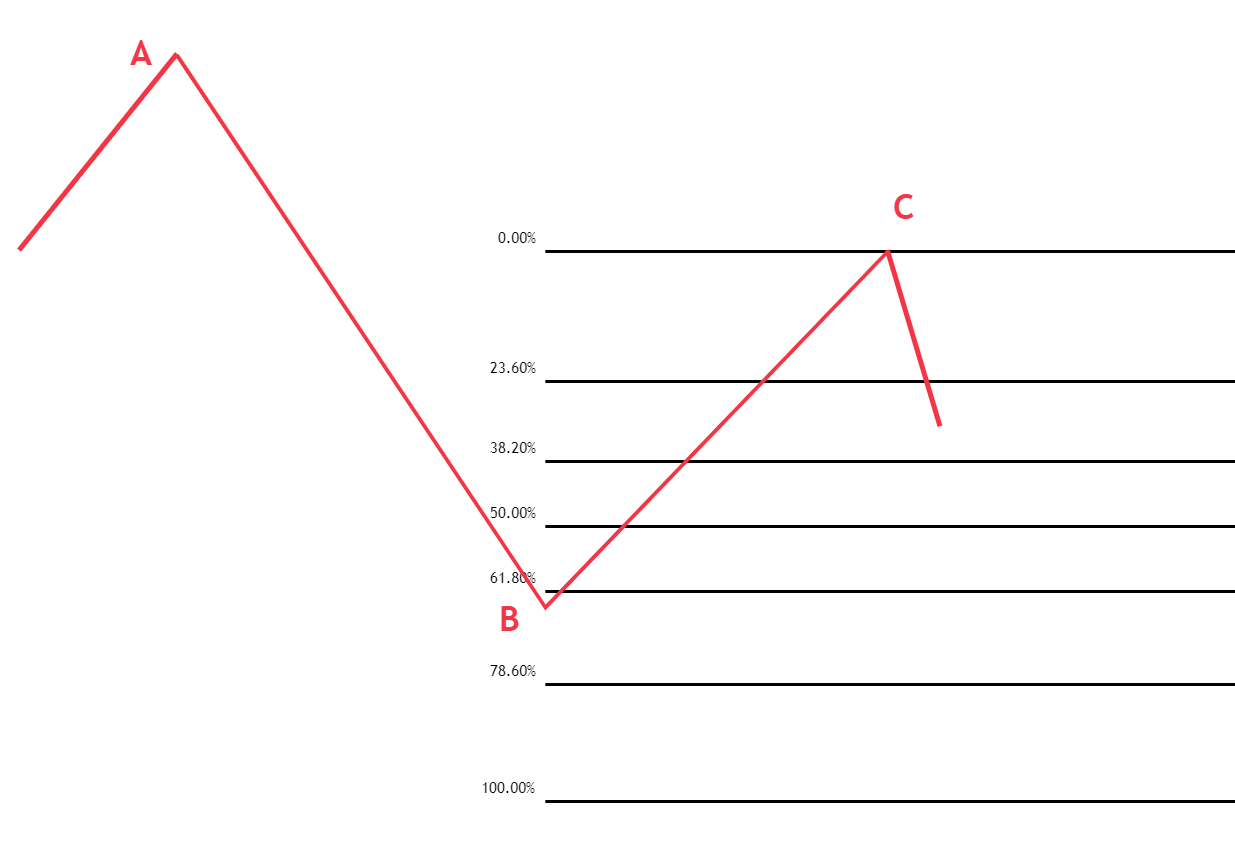##### Why does Fibonacci Work?

A lot of traders puts alerts at different Fibonacci retracement levels and look for trade opportunities at a better price

1. The price action trader also looks for buy-on dips in established uptrends
2. And any other indicator trader also looks for entries like a trendline. moving average etc

All these confluence factors increase the probability of success

##### What is the Fibonacci retracement trading strategy?

Area of support in the uptrend and area of resistance in the uptrend. The fundamental idea behind the Fibonacci retracement trading strategy.  states that after beginning a new trend direction, the price will retrace before continuing in the trend’s direction. Therefore, we utilize the Fibonacci tool to identify the following probable support and resistance levels. The maximum level at which retracement is likely to occur is predicted by the retracement level. Traders have an excellent opportunity to start new trades in the trend’s direction at these retracement levels. The Fibonacci retracement levels are 23.6%, 38.2%, 50%,61.8%, 78.6%, and 100%.

Next possible support levels are marked if the stock moves up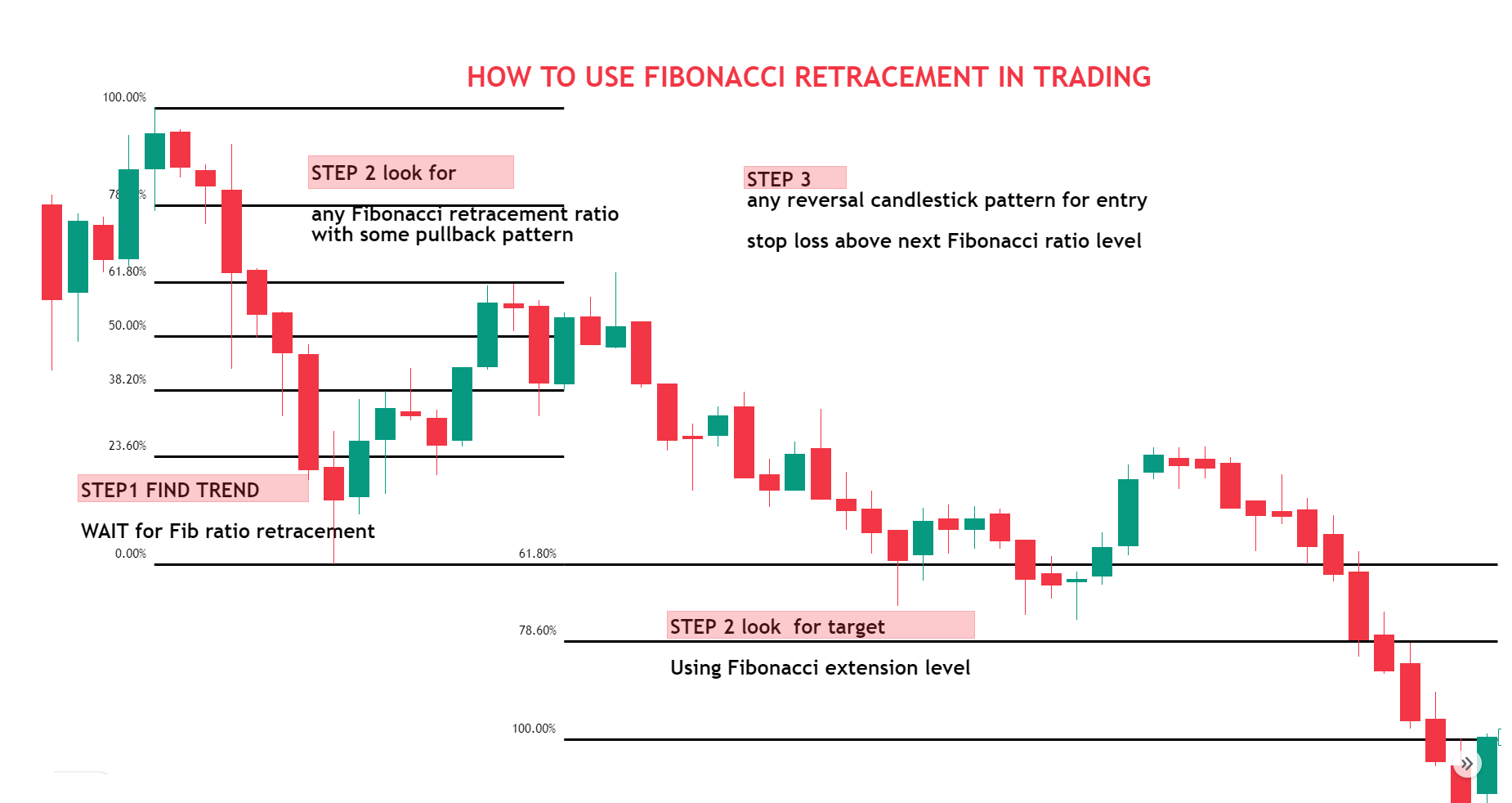#### Fibonacci Trading Strategy with Confluence

##### What is the Area of Confluence?

Confluence means finding multiple reasons for taking a trade. “Area of Confluence” in trading refers to a certain price zone or level when several technical analysis tools and indicators converge and suggest the same possible price movement. Confluence zones are considered stronger levels of support or resistance since the interaction of several parameters adds more support to prospective price action. Many types of traders take entry at a zone with the same view which creates momentum in your favor.

The Fibonacci with confluence factor trading strategy involves combining the use of Fibonacci retracement levels with various other parameters to identify potential trade opportunities.

##### Finding Area of Confluence in Fibonacci Trading Strategy

Here are some confluence factors that can use along with the Fibonacci retracement trading strategy

1. Fibonacci retracement
2. Horizontal support and resistance
3. Supply and demand zone
4. Trendline
5. Moving average
6. Chart pattern

More the confluence stronger the signal

2. Clear impulse move
3. The first pullback in a newly established trend
##### Fibonacci Trendline Confluence Trading Strategy

The Fibonacci with trendline trading strategy involves combining the use of Fibonacci retracement levels with trendlines to identify potential trade opportunities in the financial markets.

A trendline is a straight line that connects two or more price points and is used to identify the direction of the current trend in a market. In the context of the Fibonacci with trendline trading strategy, trendlines are used to confirm the direction of the trend and to determine the validity of the Fibonacci retracement levels.

###### Here is how the strategy works:
1. Identify the trend: Search for an instrument that is either rising (higher highs and higher lows) or falling (lower highs and lower lows)
2. Draw a trendline: Draw a line on the chart that connects two or more lows (for an uptrend) or two or more highs (for a downtrend) to indicate the trend. You can use the trendline as a level of support or resistance to assist you to decide whether to buy or sell.
3. use the Fibonacci retracement tool to find out key levels of retracement and look for the confluence of both trendlines with the Fibonacci retracement level
4. Identify entry and exit points: If the price is in an uptrend and above the trendline, consider buying near the support of the trendline. Consider selling at the trendline resistance if the price is below the trendline and the trend is downward.
5. Stop loss and target: – Put stop-loss orders below the trendline in an uptrend or above the trendline in a downturn to reduce risk. Additionally, consider using profit objectives to maximize profits at predefined levels.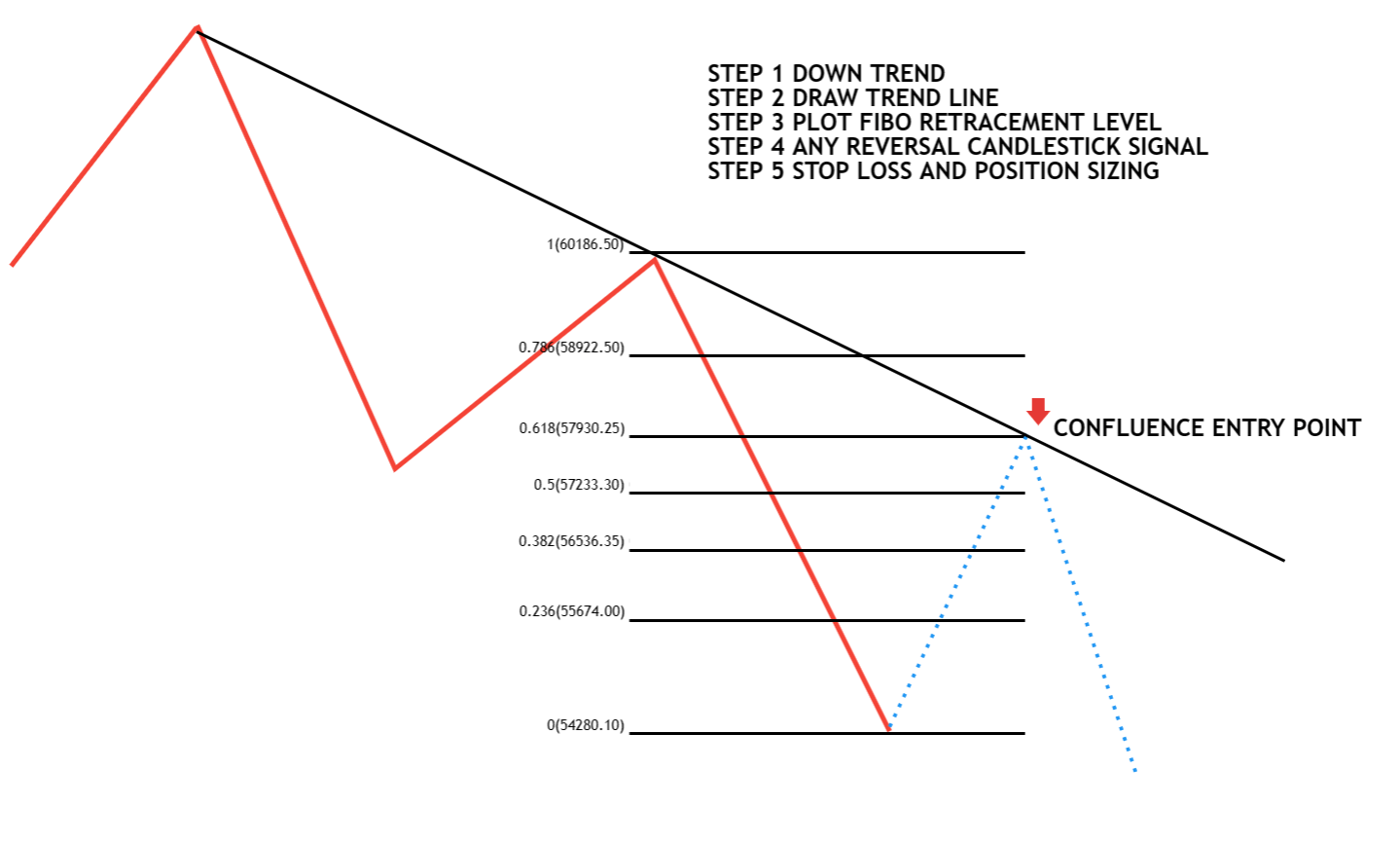BELOW IS AN EXAMPLE OF A BANK NIFTY DAILY TIME FRAME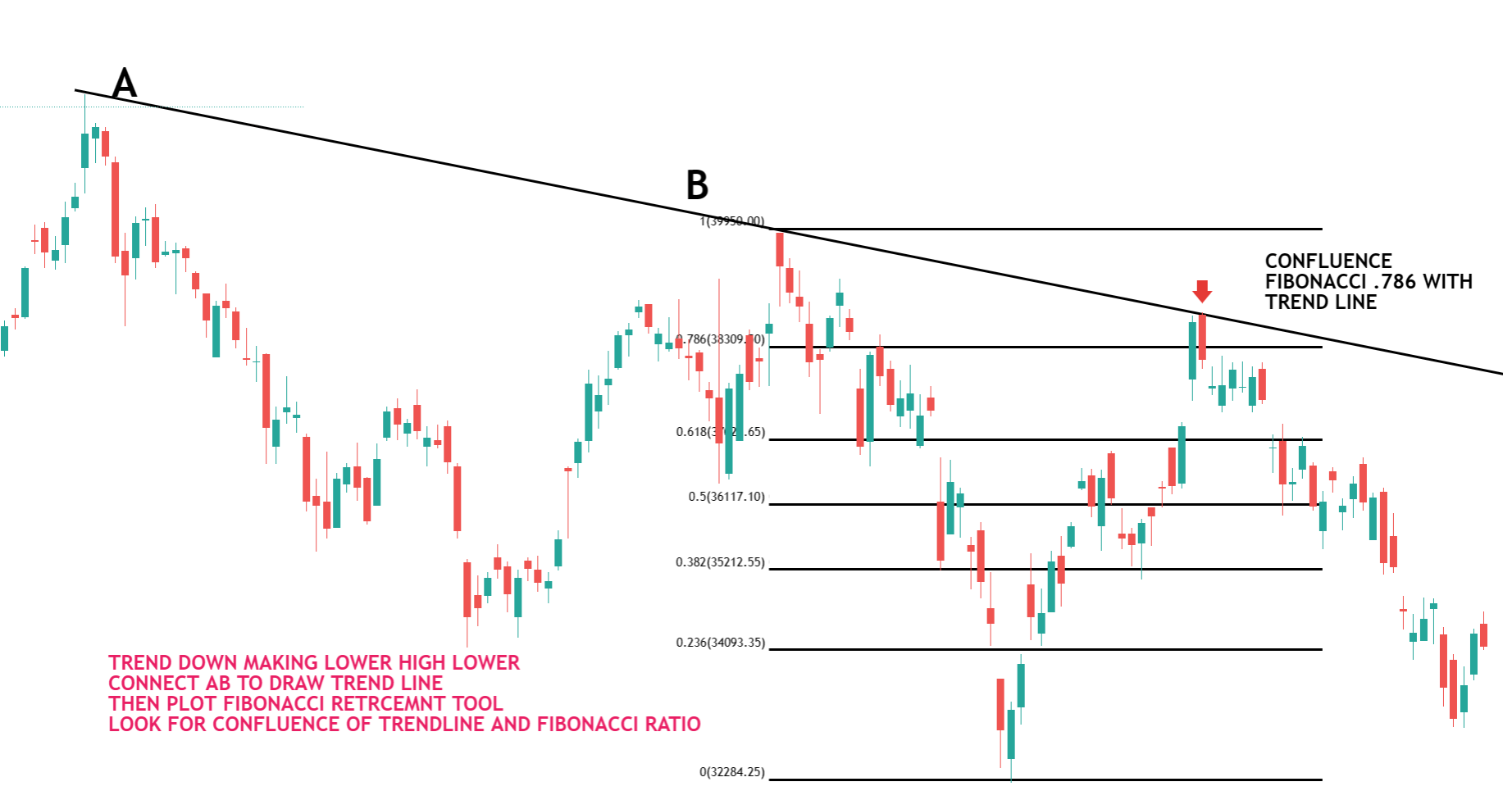##### Fibonacci with Support and Resistance or Supply and Demand Zone Confluence Trading Strategy:

In order to find suitable entry and exit opportunities, many traders combine the confluence of Fibonacci retracement levels with support and resistance levels or supply and demand zone. In order to pinpoint places where the price may reverse, this method combines Fibonacci retracement levels with significant support and resistance levels.

With this approach, traders look for confluence between important support and resistance levels or supply and demand zone and Fibonacci retracement levels.

Here is a step-by-step guide on how to use the confluence of Fibonacci retracement levels with support and resistance levels

1. The first step is to identify a clear trend in the market. This can be done by looking at the price action. means there should be a clear impulse to move beyond support resistance.
2. The second step is to identify key levels of support and resistance or supply and demand zone in a clear trend. This can be done by looking for previous price levels where the price has breakout or reversed
3. The third step is to use the Fibonacci retracement tool to find out the key level of retracement and look for the confluence of both support or resistance with the Fibonacci retracement level
4. Identify entry and exit points: If the price is in an uptrend consider buying near the support of the trendline
5. Stop loss and target: – ABOVE ENTRY SIGNAL HIGH IN DOWN TREND.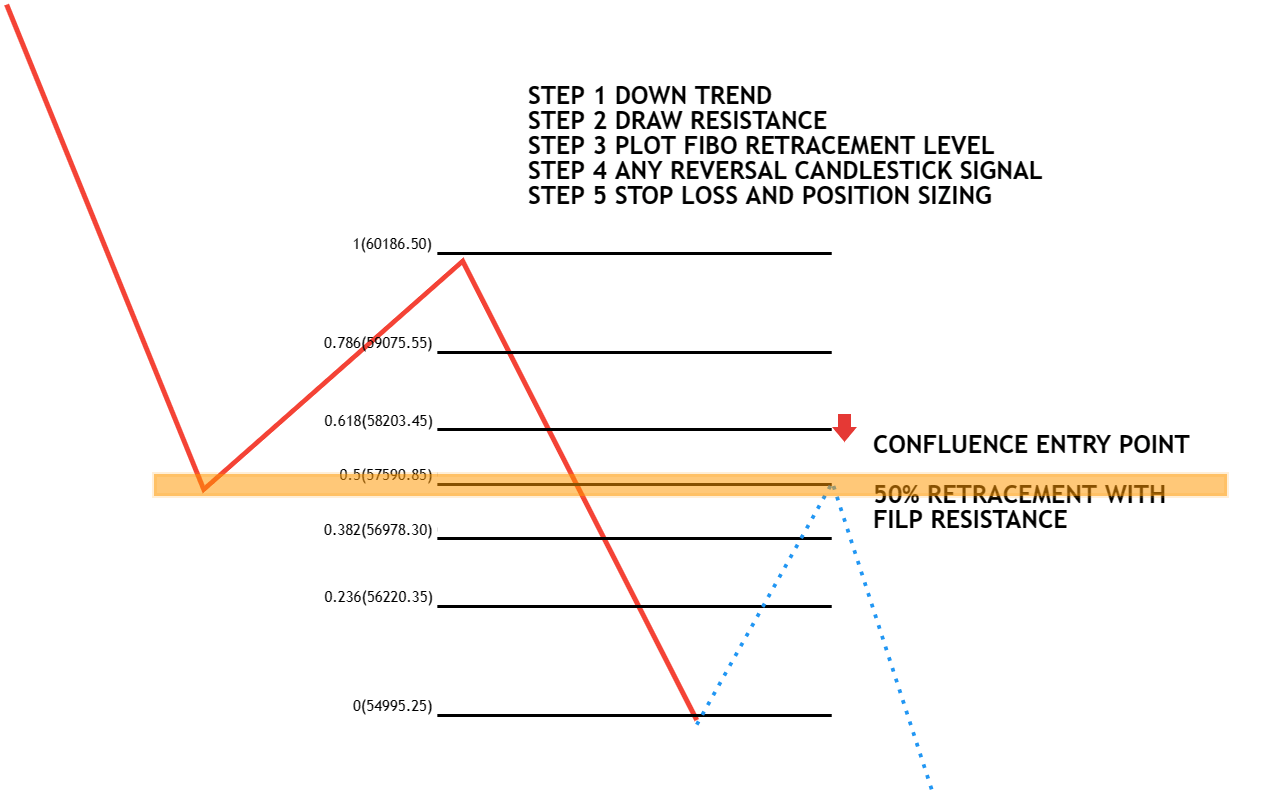Here is an example of a nifty 2 hours time frame.505 Fibonacci retracement with flip resistance and then a downtrend continued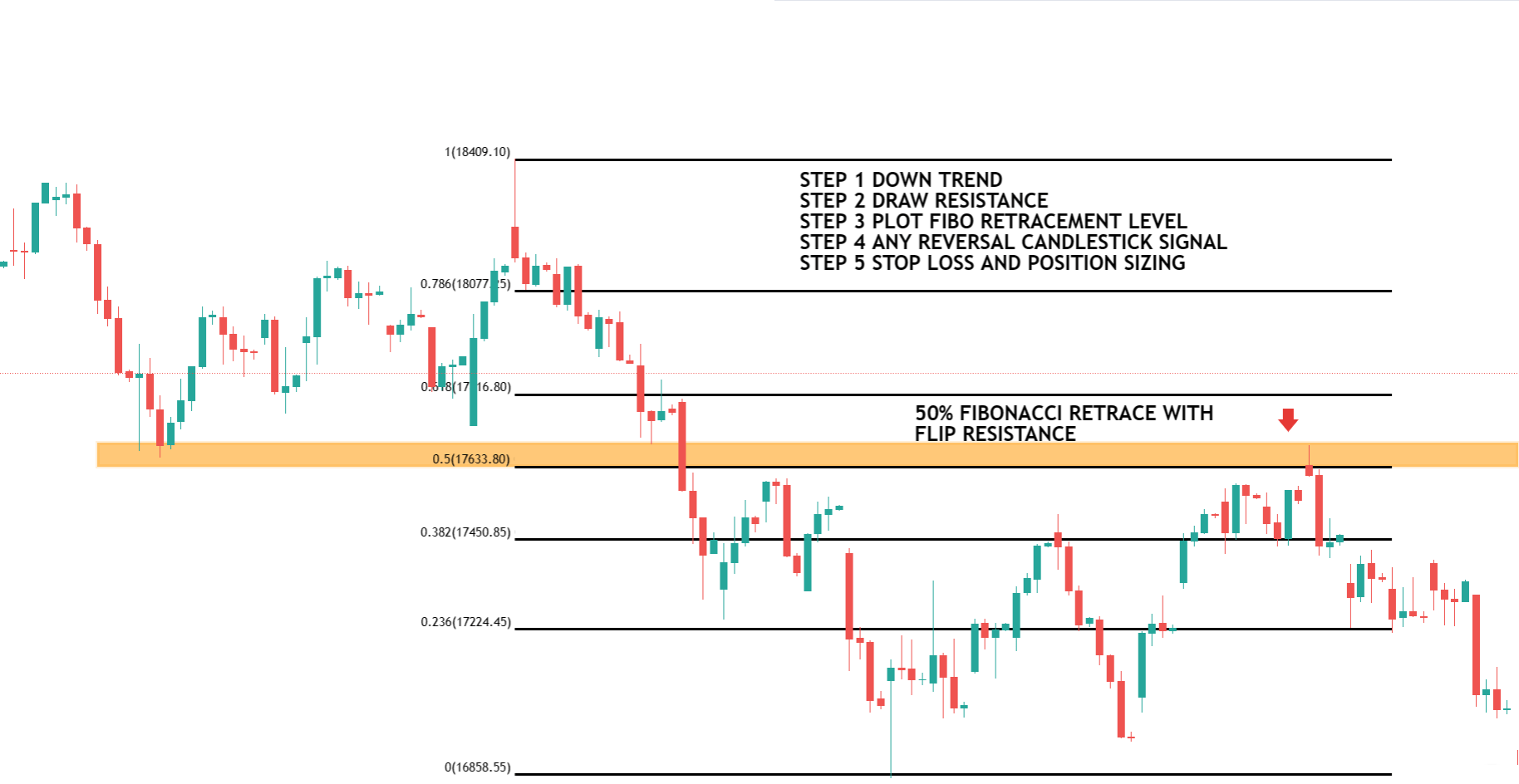Below is the example of Fibonacci with a supply and demand zone and then a downtrend continued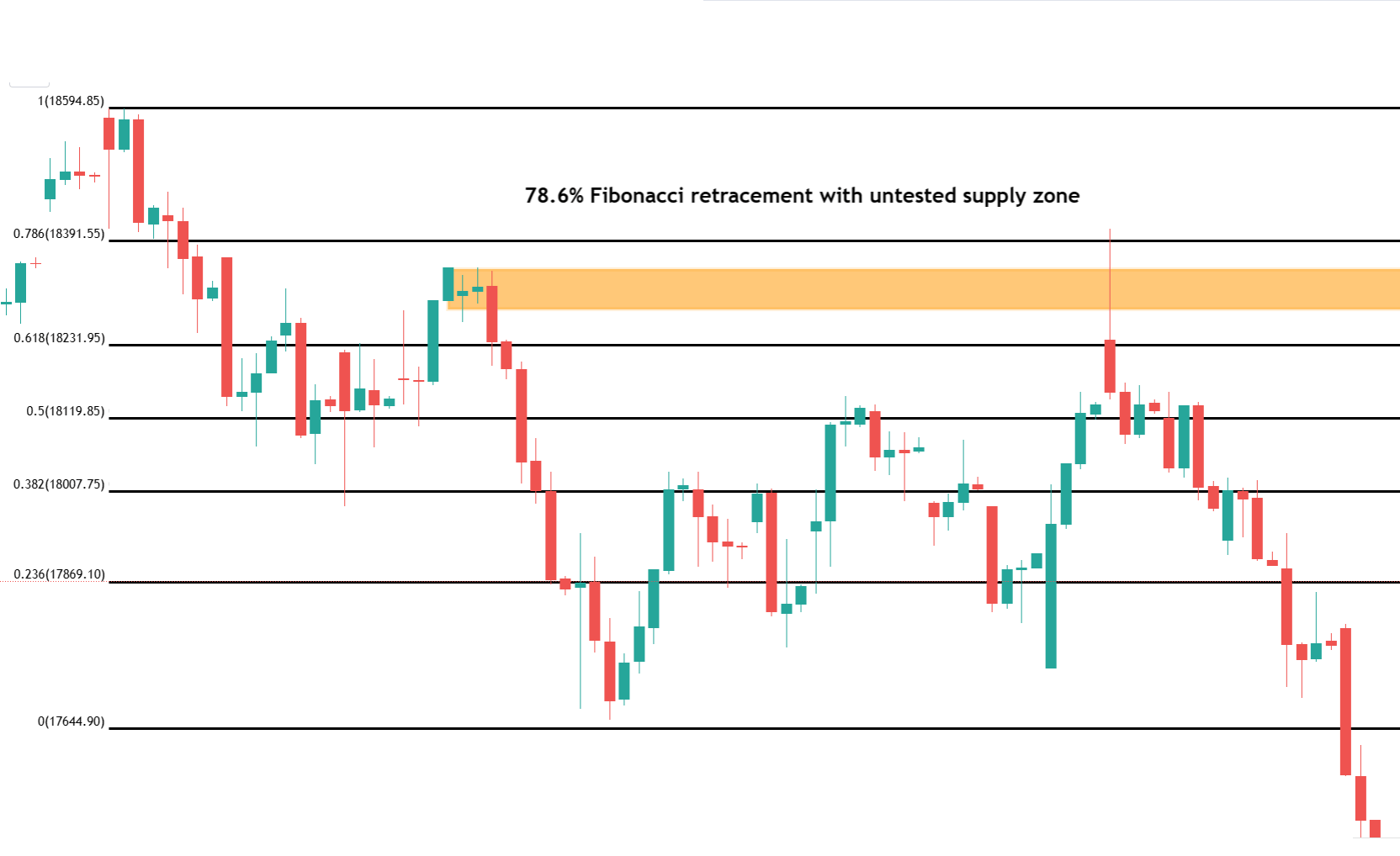##### Fibonacci with Moving Average Confluence Trading Strategy:

Using two well-known indicators, the Fibonacci plus moving average confluence trading technique can spot probable market trends and reversals. Traders look for confluence between these two indicators to confirm their trading decisions, and the strategy is popular among technical analysts for its accuracy in identifying potential trading opportunities.

1. Plot moving averages on your chart. Moving averages can help to identify the direction of the trend and provide potential areas of support and resistance.
2. Use the Fibonacci retracement tool to plot the Fibonacci levels between a high and low point in the trend.
3. look for confluence between the Fibonacci levels and the moving averages. If a key moving average aligns with a key Fibonacci retracement level, such as the 61.8% or 50% retracement, this creates a stronger area of support or resistance.
4. if you find a confluence between the Fibonacci levels and the moving averages, the next step is to make a trade decision. For example, if you find a confluence of support at the 61.8% Fibonacci retracement level and a 50-moving average, you may decide to enter a long position at that level

BELOW IS AN EXAMPLE OF A DAILY TIME FRAME IN NIFTY. HERE THE confluence of 61.8% Fibonacci retraces with flip support and 50 simple moving average. then the price continues with the trend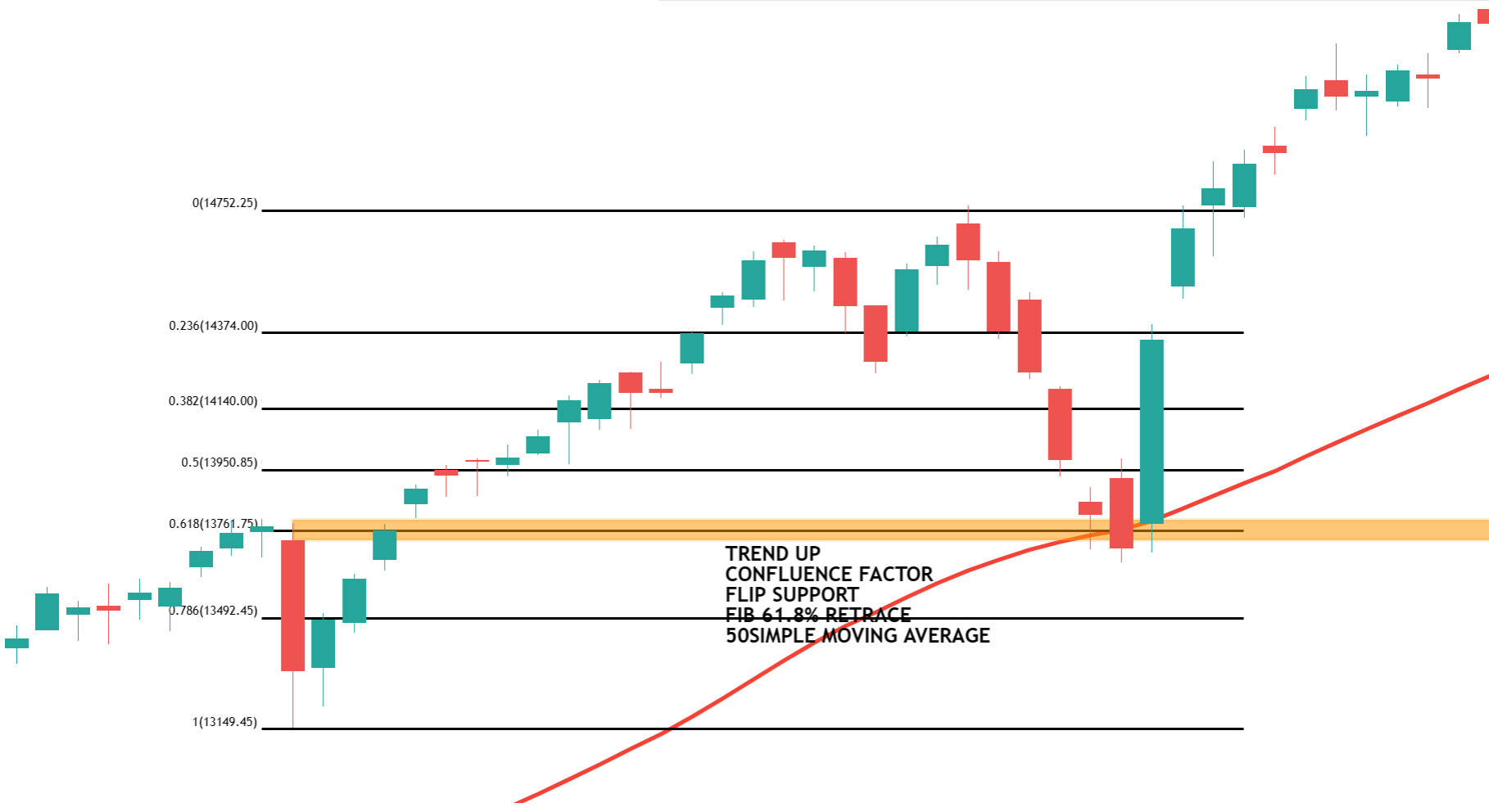##### Fibonacci Trading Strategy with Multiple Confluence Factors

The Fibonacci with multiple confluence methods uses a variety of indicators to determine possible places of support and resistance for entry and exit in the market.

finding important Fibonacci retracement levels and combining them with other confluence factors like moving averages, trend lines, support and resistance levels, supply and demand zones, and chart patterns. Traders can get a more complete picture of the market and potentially find more precise trading signals

Let us see another example of the nifty daily time frame and also the extension of the previous chart. Here the confluence factor falling wedge, 100 simple moving average with 61.8% Fibonacci retrace also one can see a double bottom reversal